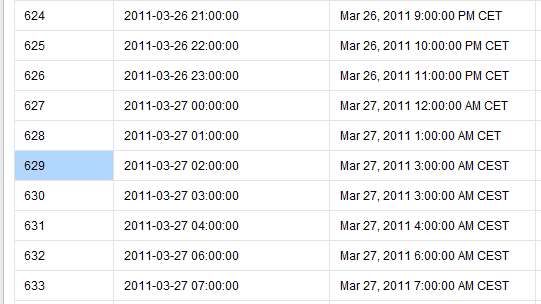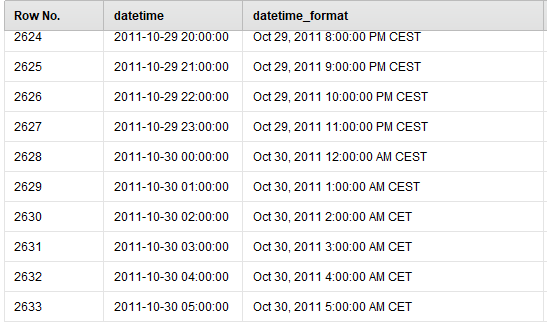# CET/CEST Datetime Format

Member Posts: 19Maven
edited December 2018 in Help

Hello,

I have a problem with date_time attribute with format yyyy-MM-dd HH:mm:ss

I can import this column from a CSV file as date_time type. No problem.

For example: 2011-03-26 23:00:00     it looks like       Mar 26, 2011 11:00:00 PM CET

using to format:

• date type: date_time
• date format: yyyy-MM-dd HH:mm:ss
• locale: Spanish

But, when I look into all the values I have found one problem when time changes from CET to CEST.

datetime (nominal type)                datetime_format (date_time type)On March 27th, the time changed from CET to CEST and then two 3 AM hours are repeated!

Then, for this row I can't do a function to return the hour because it's wrong - Using Generate Attribute operator with date_get(datetime_format,DATE_UNIT_HOUR) -

To get the correct hour I have used datetime (as a nominal type) and using a function to obtain the hour - Using Generate Attribute operator with cut(datetime,11,2).

Any other suggestion to solve this?

There is no problem changing from CEST to CETAll the best,

Montse

• Moderator, RapidMiner Certified Analyst, RapidMiner Certified Expert, Member Posts: 1,635Unicorn
Solution Accepted

This whole thread is just a reminder to me why I hate time zone changes!  Things would be much easier if we would all pick a time offset from UTC and stick with it.

Montse, in the original date series, there is an entry for 2am and 3am on the day that the clocks switch. As Scott pointed out, there is no 2am that day, so is every entry after that really off by one hour (e.g., did the mechanism that captured the time interval not realize that on that particular day because of the time switch there would be only 23 hourly intervals and not 24)?

Brian T.
Lindon Ventures
Data Science Consulting from Certified RapidMiner Experts

• Administrator, Moderator, Employee, RapidMiner Certified Analyst, Community Manager, Member, University Professor, PM Moderator Posts: 2,959Community Manager

hello @Montse - ok that is a super-interesting question (at least to me).

So I think RapidMiner is 100% correct. There is no such thing as 02:00 on March 27, 2011. It literally does not exist. After 01:59:59 the clocks immediately flip to 03:00:00 :

01:59:58

01:59:59

03:00:00

03:00:01

etc...

So if you ask RapidMiner to give you 02:00 CEST and 03:00 CEST, they MUST be the same. It is the same time of day.As for how to cope with this in your file, if it were me I would remove duplicates as the data in 629 and 630 must be the same.

ooh great question!

Scott

• Administrator, Moderator, Employee, RapidMiner Certified Analyst, RapidMiner Certified Expert, University Professor Posts: 3,453RM Data Scientist

Hi,

just as a reminder: Dates in RM are always stored as ms since 1970. Whatever is shown in the ExampleSet for date_time attributes is only a visualization layer on it.

It gets tricky if you convert back and forth to nominal though.

BR,

martin

- Sr. Director Data Solutions, Altair RapidMiner -
Dortmund, Germany
• Member Posts: 19Maven

Hi @sgenzer and @mschmitz,

The file has not duplicate datetimes columns, as you can see from the attached file.

When I load the data into date_time format is when appears these duplicates, when RapidMiner changes from CET to CEST (in visualization layer as  @mschmitz says). And then, when I try to get the hour from this datetime column (with date_time format, not nominal) using the date_get function is when appears the error.

All the best,

Montse

test.csv 316.3K
• Member Posts: 19Maven

Thank you, Brian.

As you have said it could be easier to try to pick the time as UTC.

Yes, you are right, there is no error about 2:00 because doesn't exist.

But if I want to extract the hour in UTC format how can I do it using a date_time column? I have set using time zone as UTC but I have the same result.

It is possible that only I can solve it by working directly with the string (using the cut function to obtain the hour)?

`<?xml version="1.0" encoding="UTF-8"?><process version="8.2.000">  <context>    <input/>    <output/>    <macros/>  </context>  <operator activated="true" class="process" compatibility="8.2.000" expanded="true" name="Process">    <process expanded="true">      <operator activated="true" class="read_csv" compatibility="8.2.000" expanded="true" height="68" name="Read CSV" width="90" x="45" y="34">        <parameter key="csv_file" value="D:\test.csv"/>        <parameter key="column_separators" value=","/>        <parameter key="date_format" value="yyyy-MM-dd HH:mm:ss"/>        <parameter key="first_row_as_names" value="false"/>        <list key="annotations">          <parameter key="0" value="Name"/>        </list>        <parameter key="locale" value="Spanish"/>        <parameter key="encoding" value="UTF-8"/>        <list key="data_set_meta_data_information">          <parameter key="0" value="datetime.true.nominal.attribute"/>          <parameter key="1" value="season.true.integer.attribute"/>          <parameter key="2" value="holiday.true.integer.attribute"/>          <parameter key="3" value="workingday.true.integer.attribute"/>          <parameter key="4" value="weather.true.integer.attribute"/>          <parameter key="5" value="temp.true.real.attribute"/>          <parameter key="6" value="atemp.true.real.attribute"/>          <parameter key="7" value="humidity.true.integer.attribute"/>          <parameter key="8" value="windspeed.true.real.attribute"/>          <parameter key="9" value="casual.true.attribute_value.attribute"/>        </list>      </operator>      <operator activated="true" class="nominal_to_date" compatibility="8.2.000" expanded="true" height="82" name="Nominal to Date" width="90" x="179" y="34">        <parameter key="attribute_name" value="datetime"/>        <parameter key="date_type" value="date_time"/>        <parameter key="date_format" value="yyyy-MM-dd HH:mm:ss"/>        <parameter key="time_zone" value="UTC"/>        <parameter key="keep_old_attribute" value="true"/>      </operator>      <operator activated="true" class="rename" compatibility="8.2.000" expanded="true" height="82" name="Rename" width="90" x="313" y="34">        <parameter key="old_name" value="datetime"/>        <parameter key="new_name" value="datetime_format"/>        <list key="rename_additional_attributes"/>      </operator>      <operator activated="true" class="generate_attributes" compatibility="8.2.000" expanded="true" height="82" name="Generate Attributes" width="90" x="447" y="34">        <list key="function_descriptions">          <parameter key="Hour_wrong" value="date_get(datetime_format,DATE_UNIT_HOUR)"/>          <parameter key="Hour_correct" value="cut(datetime_old,11,2)"/>        </list>      </operator>      <connect from_op="Read CSV" from_port="output" to_op="Nominal to Date" to_port="example set input"/>      <connect from_op="Nominal to Date" from_port="example set output" to_op="Rename" to_port="example set input"/>      <connect from_op="Rename" from_port="example set output" to_op="Generate Attributes" to_port="example set input"/>      <connect from_op="Generate Attributes" from_port="example set output" to_port="result 1"/>      <portSpacing port="source_input 1" spacing="0"/>      <portSpacing port="sink_result 1" spacing="0"/>      <portSpacing port="sink_result 2" spacing="0"/>    </process>  </operator></process>`

All the best,

Montse

• Moderator, RapidMiner Certified Analyst, RapidMiner Certified Expert, Member Posts: 1,635Unicorn

If you simply want the Generate Attributes version from the date attribute to match the version you extract from the string, just set the locale to English and the timezone to Eastern Time (America/New York).  See the attached process.  Now the two attributes always match.  You still have the missing hour on 3/27 at 5am (see rows 630 and 631) but there is nothing you can do about that as we discussed before!

`<?xml version="1.0" encoding="UTF-8"?><process version="8.2.000">  <context>    <input/>    <output/>    <macros/>  </context>  <operator activated="true" class="process" compatibility="8.2.000" expanded="true" name="Process">    <process expanded="true">      <operator activated="true" breakpoints="after" class="read_csv" compatibility="8.2.000" expanded="true" height="68" name="Read CSV" width="90" x="45" y="34">        <parameter key="csv_file" value="C:\Users\brian\Downloads\test.csv"/>        <parameter key="column_separators" value=","/>        <parameter key="date_format" value="yyyy-MM-dd HH:mm:ss"/>        <parameter key="first_row_as_names" value="false"/>        <list key="annotations">          <parameter key="0" value="Name"/>        </list>        <parameter key="time_zone" value="US/Eastern"/>        <parameter key="locale" value="English"/>        <parameter key="encoding" value="UTF-8"/>        <list key="data_set_meta_data_information">          <parameter key="0" value="datetime.true.nominal.attribute"/>          <parameter key="1" value="season.true.integer.attribute"/>          <parameter key="2" value="holiday.true.integer.attribute"/>          <parameter key="3" value="workingday.true.integer.attribute"/>          <parameter key="4" value="weather.true.integer.attribute"/>          <parameter key="5" value="temp.true.real.attribute"/>          <parameter key="6" value="atemp.true.real.attribute"/>          <parameter key="7" value="humidity.true.integer.attribute"/>          <parameter key="8" value="windspeed.true.real.attribute"/>          <parameter key="9" value="casual.true.attribute_value.attribute"/>        </list>      </operator>      <operator activated="true" class="nominal_to_date" compatibility="8.2.000" expanded="true" height="82" name="Nominal to Date" width="90" x="246" y="34">        <parameter key="attribute_name" value="datetime"/>        <parameter key="date_type" value="date_time"/>        <parameter key="date_format" value="yyyy-MM-dd HH:mm:ss"/>        <parameter key="time_zone" value="America/New_York"/>        <parameter key="keep_old_attribute" value="true"/>      </operator>      <operator activated="true" class="rename" compatibility="8.2.000" expanded="true" height="82" name="Rename" width="90" x="380" y="34">        <parameter key="old_name" value="datetime"/>        <parameter key="new_name" value="datetime_format"/>        <list key="rename_additional_attributes"/>      </operator>      <operator activated="true" class="generate_attributes" compatibility="8.2.000" expanded="true" height="82" name="Generate Attributes" width="90" x="514" y="34">        <list key="function_descriptions">          <parameter key="Hour_wrong" value="date_get(datetime_format,DATE_UNIT_HOUR)"/>          <parameter key="Hour_correct" value="cut(datetime_old,11,2)"/>        </list>      </operator>      <connect from_op="Read CSV" from_port="output" to_op="Nominal to Date" to_port="example set input"/>      <connect from_op="Nominal to Date" from_port="example set output" to_op="Rename" to_port="example set input"/>      <connect from_op="Rename" from_port="example set output" to_op="Generate Attributes" to_port="example set input"/>      <connect from_op="Generate Attributes" from_port="example set output" to_port="result 1"/>      <portSpacing port="source_input 1" spacing="0"/>      <portSpacing port="sink_result 1" spacing="0"/>      <portSpacing port="sink_result 2" spacing="0"/>    </process>  </operator></process>`
Brian T.
Lindon Ventures
Data Science Consulting from Certified RapidMiner Experts